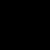## 一个很难理解的正则表达式

"/^([0-9A-Za-z\\-_\\.]+)@([0-9a-z]+\\.[a-z]{2,3}(\\.[a-z]{2})?)\$/i"

"\." 表示匹配一个无意义的点;

0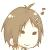\\第一个斜杠表示php里的转义，第二个斜杠表示正则里的转义。

\-表示正则里面的转义，-在正则里表示从“A到C”中的“到”，有些情况下会被误解析，所以加个转义到这表示横杠字符的本义。

\.表示匹配一个.本身这个字符，前面的\表示正则里的转义。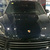0

0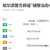.....

0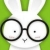EMAIL验证是这个表达试

^\w+([-+.]\w+)*@\w+([-.]\w+)*\.\w+([-.]\w+)*\$

0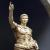`const pattern =  "#\{(?:(?<name>\w+):(?<paras>(?:\w+='[\w|\/|\s]+',?)*))(?:/\}|\}(?<snippet>(?:[^{]*|(?!\{\w+:\})++|(?R))*+)\{end\})#mu";`

-1